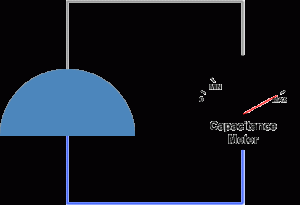What are air capacitors? Air capacitors are capacitors which use air as their dielectric. The simplest air capacitors are made of two conductive plates separated by an air gap. Air capacitors can be made in a variable or fixed capacitance form. Fixed capacitance air capacitors are rarely used since there are many other types with superior characteristics. Variable air capacitors are used more often because of their simple construction. They are usually made of two sets of semicircular metal plates separated by air gaps. One set is fixed and the other is attached to a shaft which allows the user to rotate the assembly, therefore changing the capacitance as needed. The larger the overlap between the two sets of plates, the higher the capacitance. The maximum capacitance state is achieved when the overlap between the two sets of plates is highest, while the lowest capacitance state is achieved when there is no overlap. Variable air capacitors are used in circumstances where the capacitance needs to be varied. They are sometimes used in resonant circuits, such as radio tuners, frequency mixers or antenna impedance matching applications. Another use for variable capacitors is while prototyping an electronic circuit design. Sometimes, it is simply easier to find the appropriate capacitor value by trial and error instead of calculating it exactly. Air capacitor definition Air capacitors are capacitors which use air as the dielectric medium located between conductive plates. Characteristics The dielectric constant value of a material is a measure of the amount of electrical energy stored in a material for a given voltage. Since capacitors are devices used to store electrical energy, higher dielectric constants are favorable. However, the dielectric value of air is approximately that of vacuum, which is the lowest dielectric constant possible for a material and equals εo = 8.854 * 10-12 [… read more]